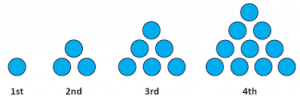# Introduction to Sequences

A sequence is an ordered set of elements – usually numbers – and is generally written with large curly brackets:

{1st number, 2nd number, 3rd number …}

For example:

{10, 9, 8, 7, 6, …}

A sequence may be finite, in which case there are a set number of terms to the sequence. The following sequence starts at 10, ends at zero and has 11 terms. It is common to show the first three and the last two terms of a sequence – or as many terms are needed to demonstrate the pattern. This sequence generally demands a loud, booming voice and a sense of dramatic expectation:

{10, 9, 8, ….,1, 0}

A sequence that continues forever is an infinite sequence. The following sequence is a famous sequence known as the Fibonacci Sequence, named after the medieval Italian mathematician, Leonardo of Pisa. A simple internet search on the Fibonacci numbers will lead to several different and fascinating topics.

{0, 1, 1, 2, 3, 5, 8, …}

## Example 1

Sequences of shapes yield interesting numerical patterns. One such sequence is the triangle numbers, which can be created using marbles or gravel or anything you have to hand.Now, you can make the second term just by adding two stones to the first term, in which case you only need three stones to illustrate the first two terms. If you make the first term, then the second term separately, you will need 4 stones.

1. How many stones do you need to make the 10th term, all on its own?
2. How many stones do you need to make the first 10 terms separately?

Did you use any shortcuts or tricks to find the answers to these two questions?

## Example 2

Another famous sequence of shapes is the Koch Snowflake. The first shape is simply an equilateral triangle. The first three shapes are illustrated in the following applet.

1. We begin with an equilateral triangle. After trisecting the edges, we add three triangles (Snowflake 2). After trisecting the new edges, we add twelve triangles (Snowflake 3). If we were to trisect the edges one more time, how many triangles would we add?
2. Suppose the first triangle has side length 9 cm. The perimeter of the first iteration is 27 cm. Then, one equilateral triangle of side length 3 cm is drawn on each side. The perimeter of the second iteration is 36 cm (Snowflake 2). Next, twelve equilateral triangles with side length 1 cm are drawn on each edge. What is the perimeter of the third iteration?

On each iteration of the Koch Curve, we get a new value for the number of triangles, the perimeter of the snowflake and for the area of the snowflake.

Understanding the mathematics of basic sequences helps to analyze the sequences that are generated by the Koch Curve.

Here are a few more iterations, by Michael Oritz:

# Why Sequences?

Sequences show up in a number of different areas of study in pure and applied math. A sequence can be found anywhere where numbers show up in an order.

In probability, you might consider how many times you need to throw a dice before you throw a six, on average – is it your first, second, third … throw? The mathematics of probability is the foundation of the success of any insurance company – car, travel, home, life insurance.

In the world of finance, you might decide to start saving now for a gap year by putting \$100 a month into a bank account. The bank will also be applying interest to your money. The mathematics of sequences allows us to calculate how much money you might save in three years.

In the study of calculus, we study the behaviour of many different types of curves. Sometimes, a sequence that behaves in the same way as a function is easier to think about, or vice versa. So we often compare curves to sequences and sequences to curves to find out more about them.